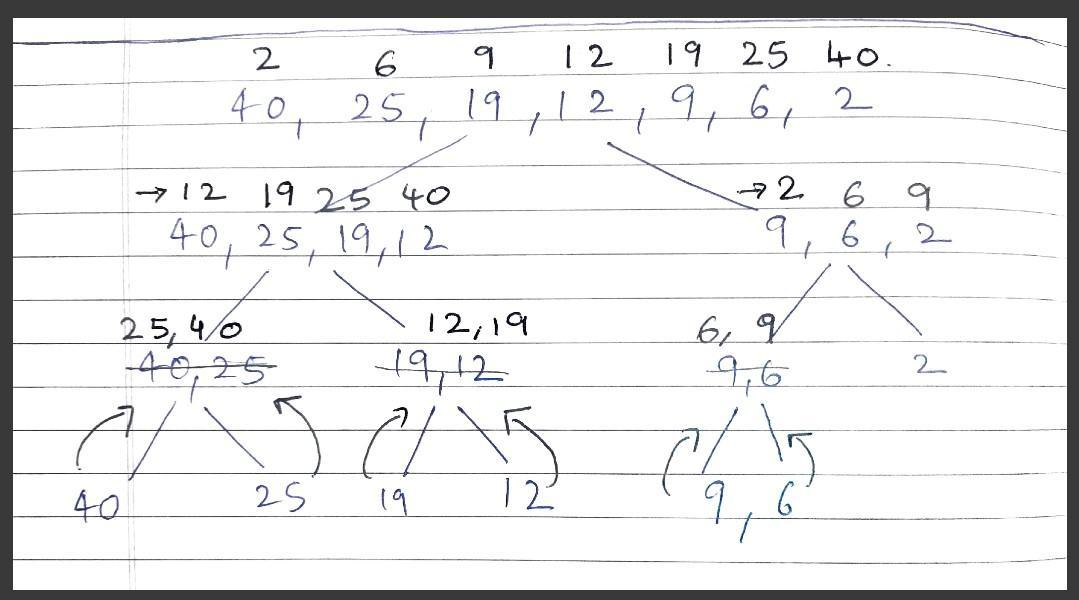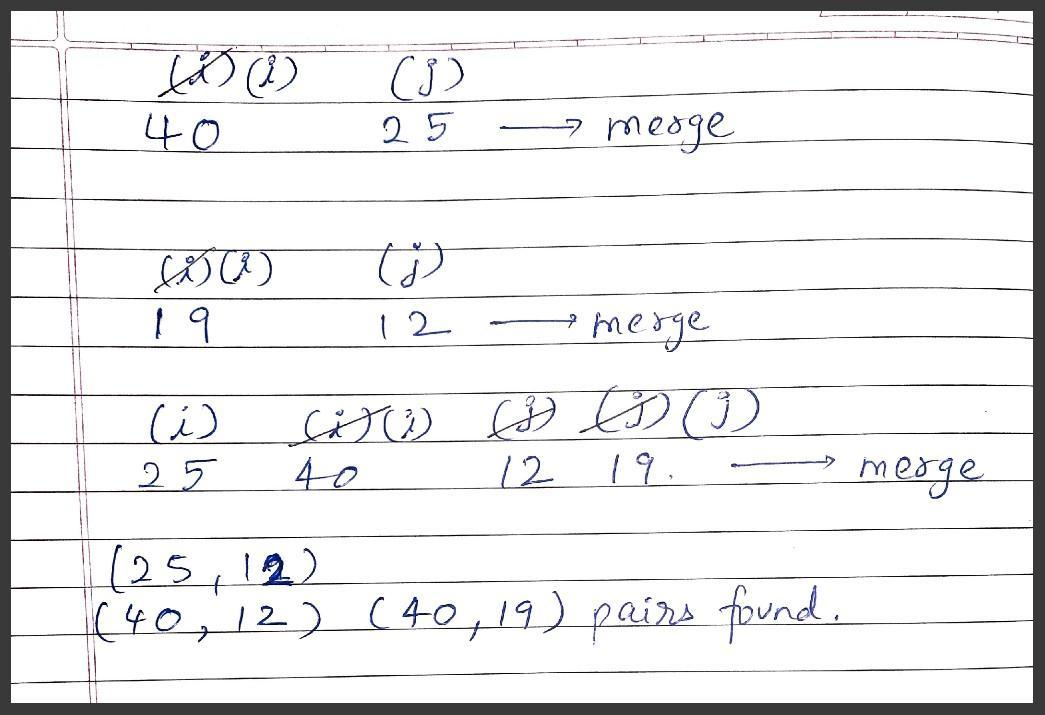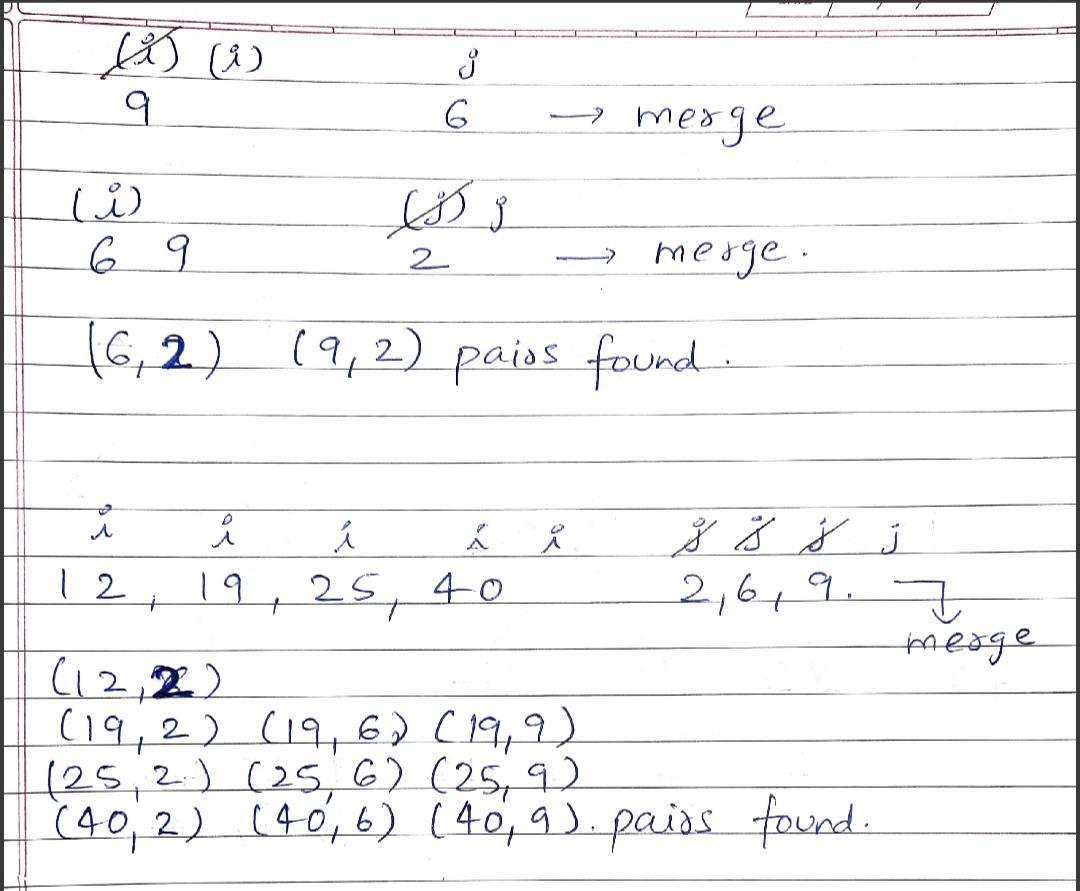# Count Reverse Pairs

Problem Statement: Given an array of numbers, you need to return the count of reverse pairs. Reverse Pairs are those pairs where i<j and arr[i]>2*arr[j].

Examples:

```Example 1:

Input: N = 5, array[] = {1,3,2,3,1)

Output: 2

Explanation: The pairs are (3, 1) and (3, 1) as from both the pairs the condition arr[i] > 2*arr[j] is satisfied.

Example 2:

Input: N = 4, array[] = {3,2,1,4}

Output: 1

Explaination: There is only 1 pair  ( 3 , 1 ) that satisfy the condition arr[i] > 2*arr[j]```

### Solution

Disclaimer: Don’t jump directly to the solution, try it out yourself first.

Solution 1: Brute Force Approach

Intuition

As we can see from the given question that i < j, So we can just use 2 nested loops and check for the given condition which is arr [i] > 2* arr[j].

Approach:

• We will be having 2 nested For loops the outer loop having i as pointer
• The inner loop with j as pointer and we will make sure that 0 <= i < j < arr.length() and also arr[i] > 2*arr[j] condition must be satisfied.

Code:

## C++ Code

``````#include<bits/stdc++.h>
using namespace std;
int reversePairs(vector < int > & arr) {
int Pairs = 0;
for (int i = 0; i < arr.size(); i++) {
for (int j = i + 1; j < arr.size(); j++) {
if (arr[i] > 2 * arr[j]) Pairs++;
}
}
return Pairs;
}

int main()
{
vector<int> arr{1,3,2,3,1};
cout<<"The Total Reverse Pairs are "<<reversePairs(arr);
}``````

Output: The Total Reverse Pairs are 2

Time Complexity: O (N^2) ( Nested Loops )

Space Complexity:  O(1)

## Java Code

`````` import java.util.*;
public class Main{
static int reversePairs(int arr[]) {
int Pairs = 0;
for (int i = 0; i < arr.length; i++) {
for (int j = i + 1; j < arr.length; j++) {
if (arr[i] > 2 * arr[j]) Pairs++;
}
}
return Pairs;
}

public static void main(String args[])
{
int arr[]={1,3,2,3,1};
System.out.println("The Total Reverse Pairs are "+reversePairs(arr));
}
}
``````

Output: The Total Reverse Pairs are 2

Time Complexity: O (N^2) ( Nested Loops )

Space Complexity:  O(1)

Solution 2: Optimal Solution

Intuition:

-> We will be using the Merge Sort Algorithm to solve this problem. We split the whole array into 2  parts creating a Merge Sort Tree-like structure. During the conquer step we do the following task :

-> We take the left half of the Array and Right half of the Array, both are sorted in themselves.

-> We will be checking the following condition arr[i] <= 2*arr[j]  where i is the pointer in the Left Array and j is the pointer in the Right Array.

-> If at any point arr[i] <= 2*arr[j] , we add 1  to the answer as this pair has a contribution to the answer.

-> If Left Array gets exhausted before Right Array we keep on adding the distance j pointer traveled as both the arrays are Sorted so the next ith element from Left Subarray will equally contribute to the answer.-> The moment when both Arrays get exhausted we perform a merge operation. This goes on until we get the original array as a Sorted array.

Approach :

-> We first of all call a Merge Sort function, in that we recursively call Left Recursion and Right Recursion after that we call Merge function in order to merge both Left and Right Calls we initially made and compute the final answer.

-> In the Merge function, we will be using low, mid, high values to count the total number of inversion pairs for the Left half and Right half of the Array.

->  Now, after the above task, we need to Merge the both Left and Right sub-arrays using a temporary vector.

-> After this, we need to copy back the temporary vector to the Original Array. Then finally we return the number of pairs we counted.Code:

## C++ Code

``````#include<bits/stdc++.h>

using namespace std;
int Merge(vector < int > & nums, int low, int mid, int high) {
int total = 0;
int j = mid + 1;
for (int i = low; i <= mid; i++) {
while (j <= high && nums[i] > 2 LL * nums[j]) {
j++;
}
total += (j - (mid + 1));
}

vector < int > t;
int left = low, right = mid + 1;

while (left <= mid && right <= high) {

if (nums[left] <= nums[right]) {
t.push_back(nums[left++]);
} else {
t.push_back(nums[right++]);
}
}

while (left <= mid) {
t.push_back(nums[left++]);
}
while (right <= high) {
t.push_back(nums[right++]);
}

for (int i = low; i <= high; i++) {
nums[i] = t[i - low];
}
}
int MergeSort(vector < int > & nums, int low, int high) {

if (low >= high) return 0;
int mid = (low + high) / 2;
int inv = MergeSort(nums, low, mid);
inv += MergeSort(nums, mid + 1, high);
inv += Merge(nums, low, mid, high);
return inv;
}
int reversePairs(vector < int > & arr) {
return MergeSort(arr, 0, arr.size() - 1);
}

int main() {
vector<int> arr{1,3,2,3,1};
cout << "The Total Reverse Pairs are " << reversePairs(arr);
}``````

Output: The Total Reverse Pairs are 2

Time Complexity : O( N log N ) + O (N) + O (N)

Reason : O(N) – Merge operation , O(N) – counting operation ( at each iteration of i , j doesn’t start from 0 . Both of them move linearly )

Space Complexity : O(N)

Reason : O(N) – Temporary vector

## Java Code

`````` import java.util.*;
public class Main{
static int merge(int[] nums, int low, int mid, int high) {
int cnt = 0;
int j = mid + 1;
for(int i = low;i<=mid;i++) {
while(j<=high && nums[i] > (2 * (long) nums[j])) {
j++;
}
cnt += (j - (mid+1));
}

ArrayList<Integer> temp = new ArrayList<>();
int left = low, right = mid+1;
while(left <= mid && right<=high) {
if(nums[left]<=nums[right]) {
}
else {
}
}

while(left<=mid) {
}
while(right<=high) {
}

for(int i = low; i<=high;i++) {
nums[i] = temp.get(i - low);
}
return cnt;
}
static int mergeSort(int[] nums, int low, int high) {
if(low>=high) return 0;
int mid = (low + high) / 2;
int inv = mergeSort(nums, low, mid);
inv += mergeSort(nums, mid+1, high);
inv += merge(nums, low, mid, high);
return inv;
}
static int reversePairs(int[] nums) {
return mergeSort(nums, 0, nums.length - 1);
}

public static void main(String args[])
{
int arr[]={1,3,2,3,1};
System.out.println("The Total Reverse Pairs are "+reversePairs(arr));
}
}
``````

Output: The Total Reverse Pairs are 2

Time Complexity : O( N log N ) + O (N) + O (N)

Reason : O(N) – Merge operation , O(N) – counting operation ( at each iteration of i , j doesn’t start from 0 . Both of them move linearly )

Space Complexity : O(N)

Reason : O(N) – Temporary ArrayList

Special thanks to Shreyas Vishwakarma for contributing to this article on takeUforward. If you also wish to share your knowledge with the takeUforward fam, please check out this article